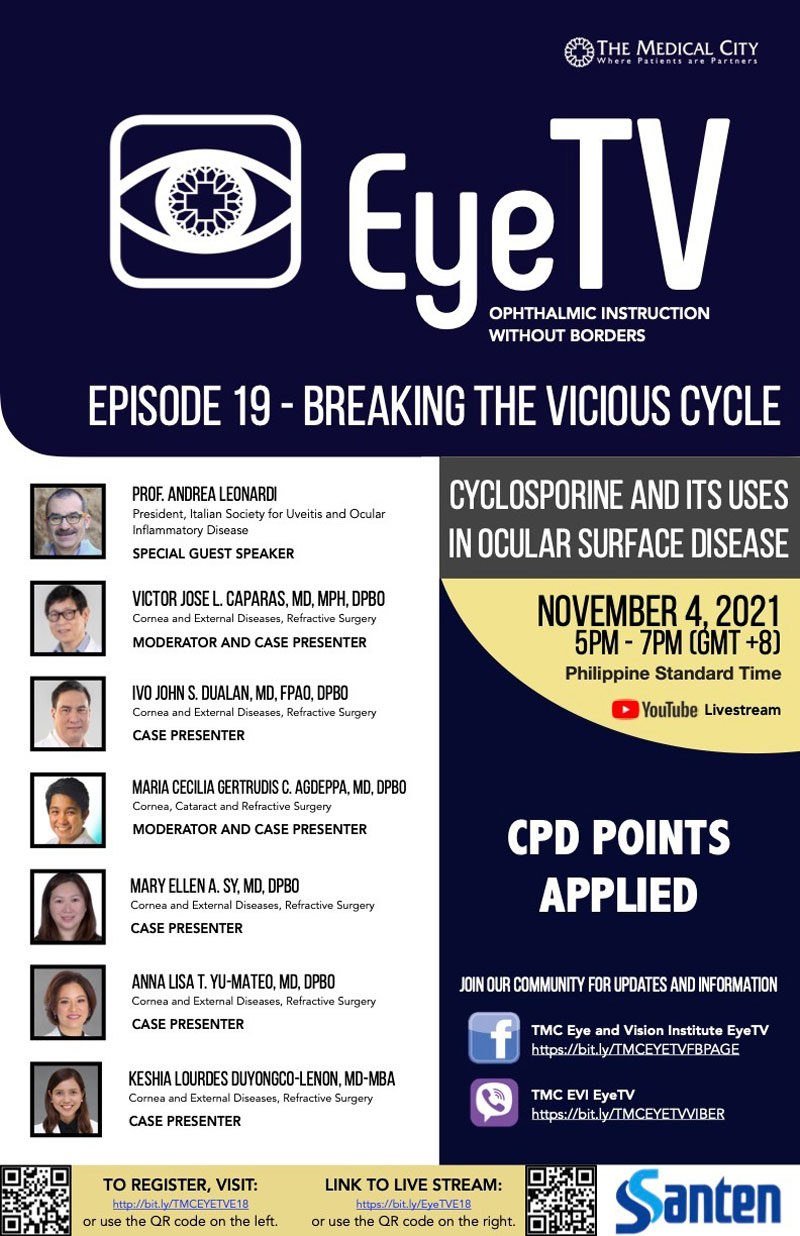Eye TV Episode 19: Breaking the Vicious Cycle``` \$(document).ready(function() { \$(".submitForm").on('click', function() { \$(this).prop('disabled', true); \$(this).css('opacity', 0.5); \$(".virnew-form-validation").html(""); if(\$("[name=firstname]").val() == "" || \$("[name=lastname]").val() == "" || \$("[name=email]").val() == "" || \$("[name=mobile]").val() == "" || \$("[name=institution]").val() == "" || \$("[name=company_position]").val() == "" || \$("[name=license_no]").val() == "" || \$("[name=date_of_expiration]").val() == "") { \$(this).prop('disabled', false); \$(".virnew-form-validation").html("Please complete all the required fields to register."); \$(this).css('opacity', 1); return false; } if(\$("[name=institution]").val() == "Select an institution*") { \$(this).prop('disabled', false); \$(".virnew-form-validation").html("Please select a province."); \$(this).css('opacity', 1); return false; } if(\$("[name=company_position]").val() == "Select a position*") { \$(this).prop('disabled', false); \$(".virnew-form-validation").html("Please select a position."); \$(this).css('opacity', 1); return false; } \$(".info").css('display', 'block'); // MODIFY this for event_page let eventPage = "eye-tv-breaking-vicious-cycle"; \$.ajax({ type: "POST", url: "https://www.virnew.com/registration/", data: { action: "eyetv19", k: "iGBLE2rS9velxwvDSQI2", event_page: eventPage, firstname: \$("[name=firstname]").val(), lastname: \$("[name=lastname]").val(), email: \$("[name=email]").val(), mobile: \$("[name=mobile]").val(), institution: \$("[name=institution]").val(), company_position: \$("[name=company_position]").val(), license_no: \$("[name=license_no]").val(), date_of_expiration: \$("[name=date_of_expiration]").val(), ref: \$("[name=ref]").val() }, success: function(r) { \$(".submitForm").css('opacity', 1); if(r == "Something went wrong." || r == "Please enter a valid email." || r == "You are already registered for this event." || r == "Sorry. Your ticket is invalid.") { \$(".submitForm").prop('disabled', false); \$(".virnew-form-validation").html(r); \$(".info").css('display', 'none'); return false; } else { window.location.href = "https://www.live.virnew.com/special_events/user-system-check/" + eventPage + "?token=" + r; return true; } }, dataType: "html" }); }); }); ```